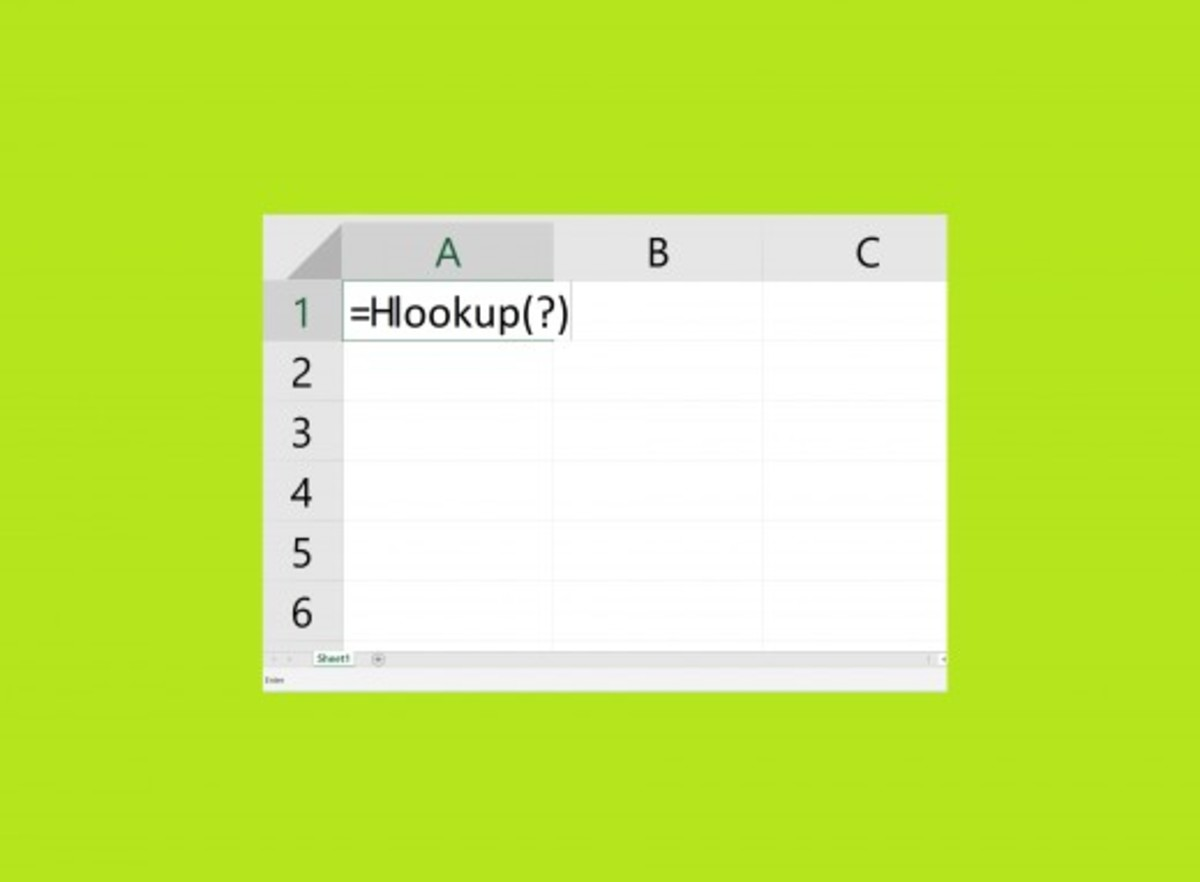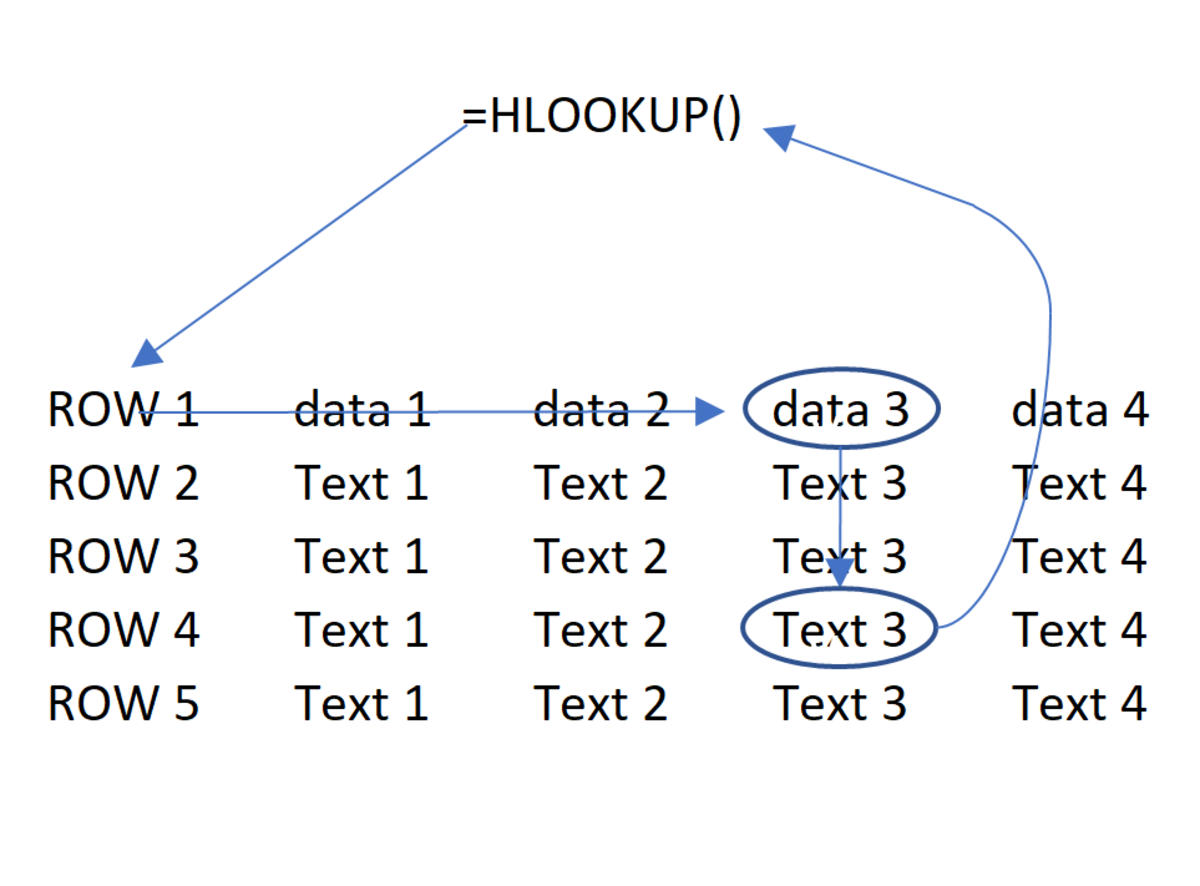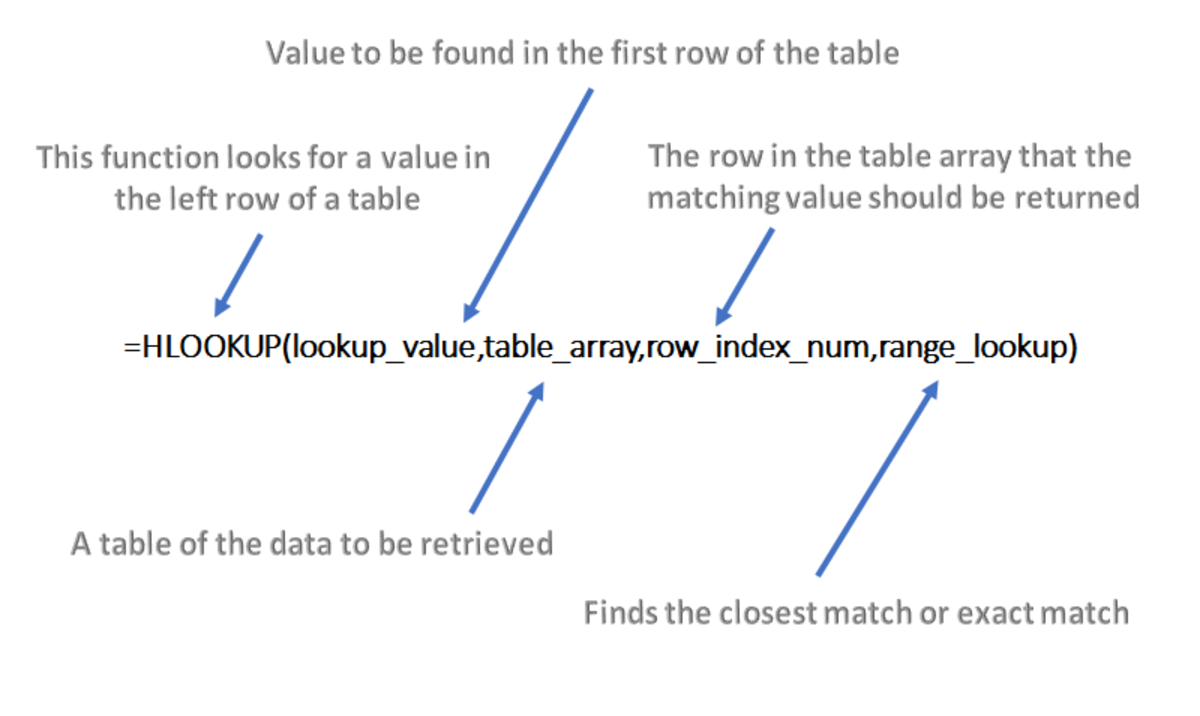Updated date:

# How to Use the HLOOKUP Function in MS Excel 2016

James likes to learn about technology and share what he learns through his articles.

## HLOOKUP FunctionThe HLOOKUP function may not be as popular as its cousin the VLOOKUP function, but still serves as an important function when certain situations prevail. This function has plenty of applications in the business world.

## Introduction

The HLOOKUP function is a Microsoft Office tool that has a lot of power considering what it can do and the amount of applications that it can be utilized for. In this article I explain the basic instructions on how use the HLOOKUP function as a lookup/reference tool for displaying information. This tutorial is explained in Microsoft Excel 2016, but can be used as reference for Excel 2007 and higher versions. If you are a beginner with Excel functions don't be fearful. This tutorial is very basic and straight forward.

## How it Works

The HLOOKUP function is useful because it can display information by using a reference value within the function. The H in HLOOKUP stands for Horizontal. When this function is utilized a piece of selected data from a row (horizontally aligned data) is referenced. After the match is made, data from a preselected row is returned to display in the cell where the function is located. An illustration of the function's process can be seen in the illustration below.

Another function that is like the HLOOKUP function is the VLOOKUP function. The V in VLOOKUP stands for vertical. The VLOOKUP has the same premise except it references information in the first column of the array to display information found in other columns.

## HLOOKUP ProcessThe HLOOKUP Excel function can be understood through this illustration. A piece of data is referenced in the first row of a table. The match is made, then a value is returned to display data that comes from a preselected a row.

## Setting Up the Function

The HLOOKUP function structure is shown below:

=HLOOKUP(lookup_value,table_array,row_index_num,range_lookup)

The first value in the function is the lookup value. Excel uses this value to search for a match in the range. The lookup value used can be a reference, number or even a formula. After the value is found in a row, Excel will can return other data to relating to that value.

The next value in the function is the table array. The table array contains row values that will be searched for and any data that may be returned to the HLOOKUP function cell. This array may be referred to as the lookup table or the lookup range.

The next value in the function is the row index number. This is the row that contains that value that you want returned to the cell that contains the function arguments. This will be inputted as a number greater than 1.

The range lookup is going to be stated as true or false. A true statement will make an approximate match when referencing the lookup table and the false statement will look for an exact match.

## Diagram of the HLOOKUP FunctionThe diagram above demonstrates the what each element of the function relates to.

## HLOOKUP Example

To become more familiar with the HLOOKUP function it's best to look at a simple example. The illustration below shows a table with student test scores, averages and a column for a letter grade on the far-right side. The reason for using a VLOOKUP here is to have a letter grade automatically appear and change as grade averages change.

## Inserting a Function

A function can be manually typed but here we will use the insert function button since this method provide some assistance to beginner if needed. To take the first step to inserting a function you must click into the cell where you need the function (letter grade) to appear. Next, click on the function tab in the main tabs section, then click on the insert function button.

## Find the Function

A window should appear titled insert function. To find the function the name or part of the name can be typed in. Go need to be selected so the function will appear on the list. Choose the function from the list and then click on the OK button.

## Second Step to Inserting a Function

Parameters of the function need to be entered one by one. starting with the lookup value. Each that parameter that was chosen for the VLOOKUP is explained below. Lookup_value

For this function the lookup value is the average. This cell is found just to the left of the cell where the function (letter grade) is. The cell only needs to be click on or its reference number can be typed in. In the example cell I5 is chosen as the lookup value.

Table_array

The table array is the table that contains the data that is being looked up. The table array chosen is D15:I16. This range contains is basically the lookup table excluding the row headers.

Row_index_num

This is the row that displays data where the function is inputted. The row that references the lookup value is always going to be row 1 so the row index must be a number greater than 1. In the example 2 is the row index and the only row that can be chosen. If there was a third row that we wanted to return data for 3 could be used.

Range_lookup

The range lookup determines whether the lookup table searches row 1 for an exact match or an approximate match. This will be set to true when an approximate match is needed and set to false when an exact match is needed. In this case an approximate match needs to be used so true will be typed into this field.

An approximate match needs to be used because the grade averages are a continuous variable and therefore there will be a very low chance of an exact match. Using true, any grade from 50 to 59 will be an D. Grades from 60 to 69 will be a C and so on.

After each parameter is entered click the OK button.

## Remove Syntax Problem

The average grade was left blank on purpose to show that a syntax problem can occur. Since no grades occur at the beginning of the semester, this function will return an F or #NA because the average column will be left blank. And no teacher or student wants to see a big fat F or an Excel error. There are a few ways around this problem but I'm going to go the path with the least resistance by using the If statement. Just below is the standard HLOOKUP that returned an F when the was no value in the average cell. In some instances, an error will occur, but the If statement will clear the error that results due to a blank cell as well.

=HLOOKUP(I5,D15:I16,2,TRUE)

To ensure an F is not returned when there is no grade an if statement is used below.

=IF(I5=" ","NG",(HLOOKUP(I5,D15:I16,2,TRUE)))

Basically, the if statement is checking to see a space (" ") in the cell and if there is it returns NG meaning "no grade." If the statement does not detect a space in the cell the it defers to the HLOOKUP function.

## Fix the Position of the Array

To utilize the fill handle to copy the HLOOKUP formula down to other records table array of the function needs to be fixed by adding dollar signs to the columns and rows of the table array. This can be completed by selecting the array and tapping the F4 key or the dollar signs can be keyed in.

## Copy the Function

After the dollar signs are added the fill handle can be used to copy the function to other records.

## Use the Fill Handle to Copy the Function

Rutkosky, N. H., Seguin, D., Davidson, J., Roggenkamp, A. R., & Rutkosky, I. (2014). Microsoft Excel 2013: Levels 1 & 2. St. Paul, MN: Paradigm Publishing.

Microsoft. (n.d.). Look up values with VLOOKUP, INDEX, or MATCH. Retrieved December 29, 2018, from https://support.microsoft.com/en-us/help/181213/how-to-use-vlookup-or-hlookup-to-find-an-exact-match

This content is accurate and true to the best of the author’s knowledge and is not meant to substitute for formal and individualized advice from a qualified professional.